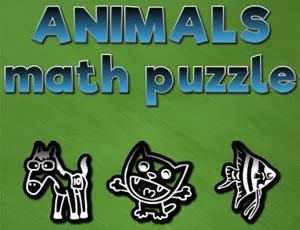## Animals math puzzlesno votes

# Animals math puzzles

Description :

Animals math puzzle is mathematical game with goal to solve 50 different mathematical problems all in limited time per task. Tasks are created as linear equations with 2 and 3 variables.
What you need to do is to find the value for the (x). (x) is a sum of the picture mark values within the (x) row. To get to (x) you need to designate a value to each picture mark according to values shown per row or column.

Game Categories :
Game tags :